# Relationship between continuous-time and discrete-time Fourier transforms

Previously in my Fourier transforms series I've talked about the continuous-time Fourier transform and the discrete-time Fourier transform. Today it's time to start talking about the relationship between these two.

Let's start with the idea of sampling a continuous-time signal, as shown in this graph: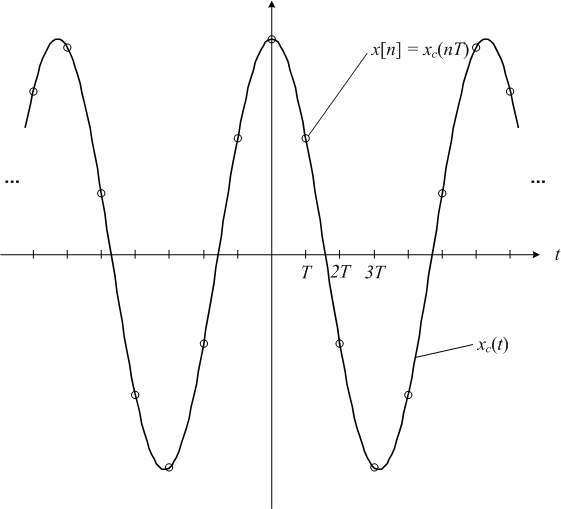Mathematically, the relationship between the discrete-time signal and the continuous-time signal is given by: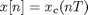(When I write equations involving both continuous-time and discrete-time quantities, I will sometimes use a subscript "c" to distinguish them.)

The sampling frequency is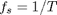(in Hz) or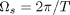(in radians per second).

The discrete-time Fourier transform of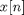is related to the continuous-time Fourier transform of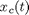as follows: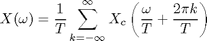But what does that mean? There are two key pieces to this equation. The first is a scaling relationship betweenand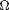: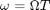. This means that the sampling frequency in the continuous-time Fourier transform,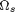, becomes the frequency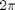in the discrete-time Fourier transform. The discrete-time frequencycorresponds to half the sampling frequency, or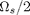.

The second key piece of the equation is that there are an infinite number of copies of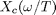spaced by.

Let's look at a graphical example. Suppose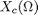looks like this: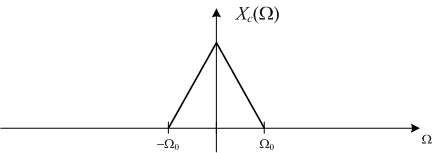Note thatequals zero for all frequencies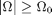. This is what we mean when we say a continuous-time signal is band-limited. The frequency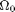is called the bandwidth of the signal.

The discrete-time Fourier transform oflooks like this: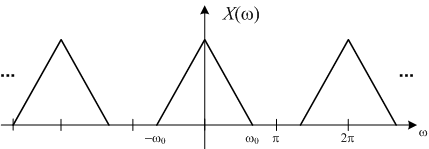where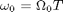. As I mentioned before, normally only one period of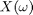is shown: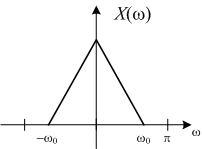For this example, then,betweenandlooks just like a scaled version of.

Next time we'll consider what happens whendoesn't look like. In other words, we're about to tackle aliasing.

Published with MATLAB® 7.9

|